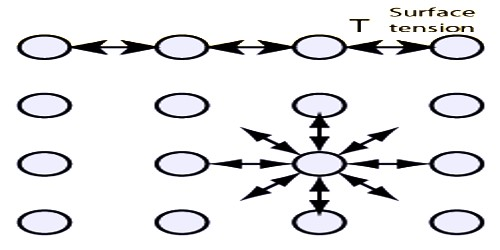# Surface Tension

Surface Tension

All liquids have a common property – liquid surface always tries to form smallest area by shrinking. The force in the liquid for which this special property is evident is called surface tension.

Definition: If a straight line is considered on the surface of a liquid, then on both sides of that line the tangential force per unit length that act on the surface of the liquid is called surface tension.

Everybody of us has noticed that mosquito, insects etc can walk on water surface. If it is observed minutely, it will be seen that where the legs touch the water surface depression appears in those places— similar to that happen when a rubber surface is pressed. Besides, when a liquid or water is passed through a syringe very slowly, it does not come out continuously, rather comes out in drops and the drops are completely spherical. We know, minimum area of the same volume is spherical shape. So, definitely there must be a force acting on the open surface that keeps the drops spherical. Hence, on the open surface of the liquid there exists a tension that acts like elastic membrane. That tension is along the tangent of the surface of the liquid. Surface tension acts on the boundary where the liquid surface ends.Unit of surface tension: Surface tension is a physical quantity. So, it has unit.

In M.K.S. and S.I. method, the unit of surface tension is Newton/metre (Nm-1).

Dimension of surface tension: We know, surface tension, T = F/L

Dimension of T = Dimension of F / Dimension of L = [MLT-1]/[L] = [MT-2]

Characteristics of surface tension: There are two important characteristics of surface tension:

(i) Surface tension tends to decrease the surface of a liquid;

(ii) Surface tension resists the increase of area of the surface of a liquid.